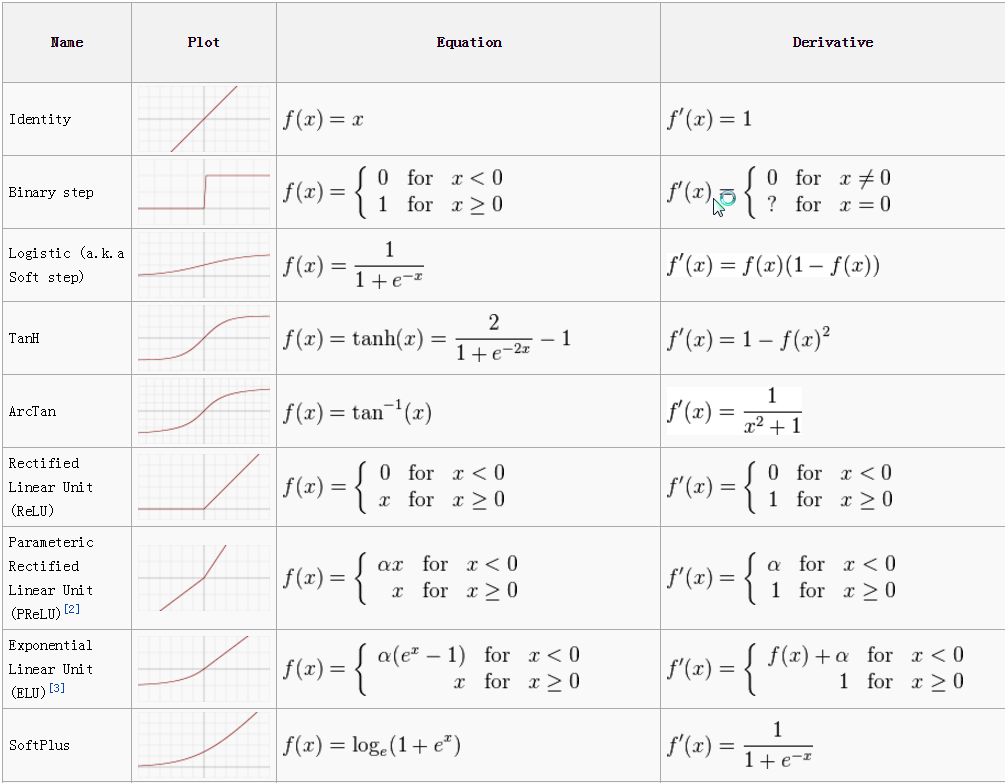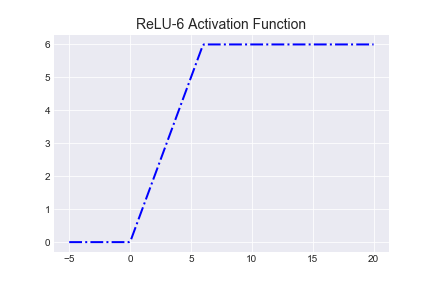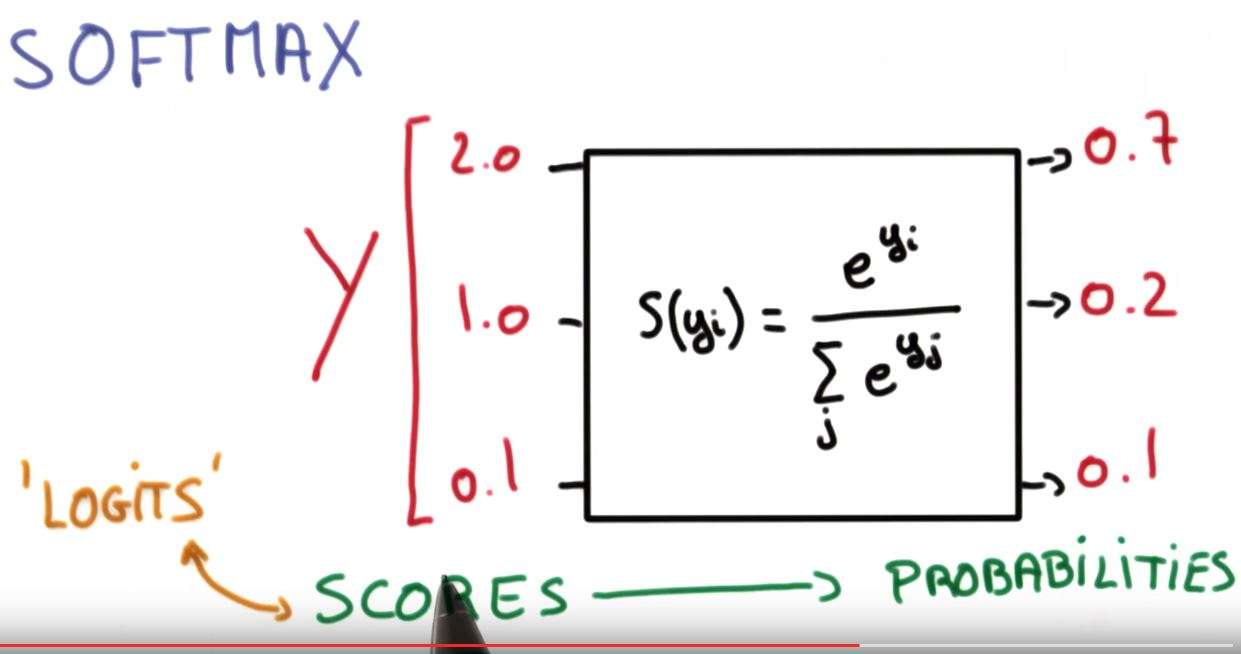### All You Need to Know about Activation Functions (Sigmoid, Tanh Relu, Leaky Relu, Softmax)

Activation functions are a crucial part of any Neural Network. A deep learning model without applying an activation function is nothing but a simple linear regression model. Activation functions map the input to the output in a particular fashion. Activation functions help us in learning the intricate structure in the dataset. Earlier, when researchers were not aware of activation functions, we were not able to make efficient use of neural networks. In this article, we will learn about the following activation functions and what are their advantages and drawbacks.

1. Linear Activation Function
2. Binary Step Activation Function
3. Sigmoid
4. Tanh
5. Relu
6. Leaky Relu
7. SoftmaxImage Source: Wikipedia

## Linear Activation Function:

In the Linear activation function, whatever input we are providing to the function same output will be generated. We can understand using the below formula.

F(x) = x (No Change in the Output)

But the problem with linear activation function is, doesn’t matter how many layers we are using in our neural network. Still, the output of our system will be linear only means our neural network will not be able to learn the non-linear structure in the dataset.

## Binary Step Activation Function:Visualization of Binary Step Function

In this activation function, we are having only two states either the output with we 0 or 1. When the input is greater than or equal to zero, the output will be one else the output will be 0.

In Binary Step Function, we can change the threshold. In the above case, we have taken the limit as 0. We can change it.

One of the significant problems with the binary step activation function is that it is non-differentiable at x = 0. So in neural networks, every time, it can not be used for classical backpropagation to update the weights.

## Sigmoid:

It is one of the commonly used activation functions in Neural Networks, also called the logistic activation function. It has an S shape. It is going to squeeze all the values in the range (0, 1). The sigmoid activation function is differentiable, so we can optimize our model using simple backpropagation. One of the most significant drawbacks with Sigmoid is it can create the vanishing gradient problem in the network as all the time gradient will be less than zero. We will learn about vanishing gradient problems in a detailed way in some other post.

## Tanh/Hyperbolic Tangent Activation Function:

It is also like Sigmoid, or even we can say that it is the scaled version of Sigmoid. Like Sigmoid, it is also differentiable at all points. Its range is (-1,1), which means given a value, it will convert the value in the range between (-1,1). As it is a non-linear activation function, it can learn some of the complex structures in the dataset. But one of the major drawbacks with it is that like Sigmoid. It also has a vanishing gradient problem because of the small value of gradients. In most of the cases, we prefer Tanh over Sigmoid.Tanh Activation FunctionDerivative of Tanh

## Relu:

It is one of the most used activation functions in 2020, and one of the states of the art activation function in deep learning. From the function, we can see that as we provide negative value to Relu, it changes it to zero; otherwise, it does not change the value. As it does not activates all the neurons at once, and output for some of the neurons is zero. It makes the network sparse and computation efficient.Derivative of Relu
But there are some of the problems with Relu as well. One of the major drawbacks is, it is not differentiable at x = 0, and at the same time, it does not have any upper bound. For some of the neurons with a negative value as input, the gradient is always zero, so the weights for those neurons do not get an update. So it may create some dead neurons, but it can be handled by reducing the learning and bias. As the mean activation in the network is not zero, there is always a positive bias in the system.

## Leaky Relu:

Relu was having one of the drawbacks that it was non-differentiable at x = 0, and if lots of negative biases are there in the data, lots of gradients will be zero, and error will not be able to propagate. It will make Relu dead. So to avoid these problems, we will use Leaky Relu.Leaky Relu Graph
In Leaky Relu, instead of making negative value directly zero, we will multiply it with some small number. Generally, this small number is .01. So in this way, we can avoid the problem of dead Relu because even if lots of negative biases are there, then also some errors will get propagated.Leaky ReluDerivative Leaky Relu
But it is not necessary that Leaky Relu outperformed Relu. Its results are not consistent. Leaky Relu should only be considered as an alternative to Relu.

## Relu-6:

In normal Relu and Leaky Relu, there is no upper bound on the positive values given to the function. But in Relu-6, there is an upper limit. Once the value goes beyond six, we will squeeze it to 6. It has been set after a lot of experiments. The upper bound encourage the model to learn sparse features early.Image Source: Google

## Softmax:

In some of the real-life applications, instead of directly getting some binary prediction, we want to know the probability of each predicted category. Softmax actually is not a classical activation function. It is generally used in the last layer of the network to provide the chances of the classes.Function for Softmax ActivationImage Source: Google
There are tons of other activation functions as well. There is no math that can tell you what activation function will work on your dataset. It’s like a hyperparameter that you need to tune using hyperparameter stunning. Here we have tried to explain the most widely used activation functions. If there is any mistake in the explanation of any activation function, please let us know in the comment section, we will try to improve it.

You May Like Some Other Articles as Well: